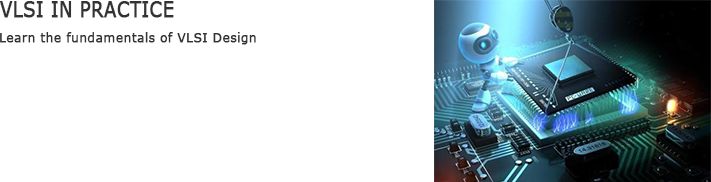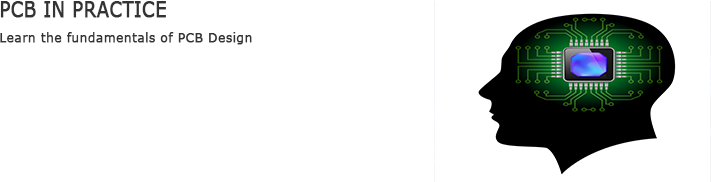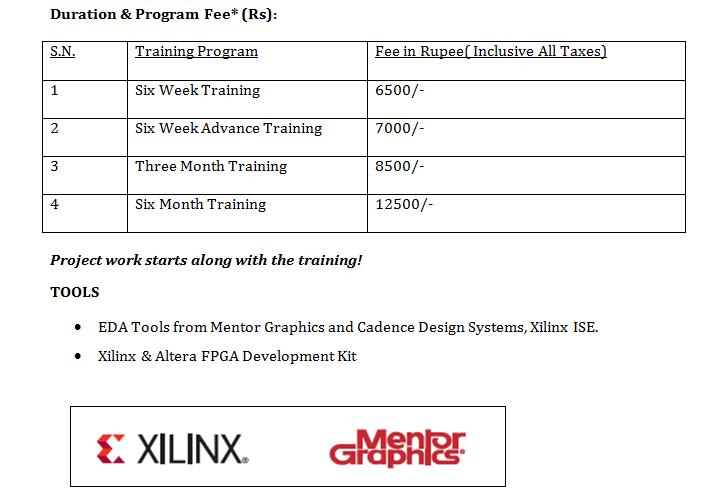VLSICOURSE For Engineering Students / COLLEGE Students## PROGRAM DETAILS:

There are different programs available in Industrial Training in VLSI Design. Students can choose the programs they are inclined to pursue.

Title Module
Introduction to VLSI
• VLSI Design Flow
• ASIC Vs FPGA
• RTL Design Methodologies
Fabrication of MOSFETs
• Introduction
• Fabrication Process Flow
• Layout Design Rules
MOS Transistor
• The MOS Structure
• Structure and operation of MOSFET
• MOSFET current and voltage characteristics
• MOSFET scaling and small geometry effects
MOS Inverters : Statics characteristics
• Introduction
• Inverters with n type MOSFET load
• CMOS Inverter
MOS Inverters: Switching Characteristics and interconnect Effect
• Introduction
• Delay Time Definitions
• Calculation of Delay Times
• Inverter Design with Delay constraints
• Estimation of Interconnect Parasites
• Calculation of Interconnect Delay
• Switching Power Dissipation of CMOS inverters
Combinational MOS Logic Circuits
• Introduction
• MOS Logic Circuits with Development nMOS Loads
• CMOS Logic Circuits
• Complex Logic Circuits
• CMOS Transmission Gate (Pass Gate)
Concepts
• Introduction
• Behavior of Bistable Element
• Voltage Bootstrapping
• Synchronous Dynamic Circuit Techniques
• High Performance Dynamic CMOS Circuits
Semiconductor memories
• Introduction
• ROM circuits
• SRAM circuits
• DRAM circuits
Low power CMOS logic circuits
• Introduction
• Overview of power consumption
• Low power design through voltage scaling
• Estimation and optimization of switching activity Capacitance